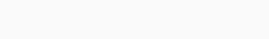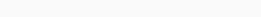# Solved – How to compute Variability Independent of the Mean (VIM)

Although I find this reading regarding the interpretation of the Coefficent of Variation (CoV) very interesting, I did not found hints regarding the Variability Independent of the Mean (VIM) which is beeing largely employed in cardiovascular research.
In particular, VIM is calculated by: $$VIM=(SD/mean^x)$$

fitting a curve through a plot of SD systolic BP (SBP; y axis)
against mean SBP (x axis) with the parameter x estimated from the
curve

Moreover, I found other different definitions/approaches:

Contents

## Computation 2:

VIM is calculated as the SD divided by the mean to the power `x` and
multiplied by the population mean to the power `x`. The power `x` is
obtained by fitting a curve through a plot of SD against mean using
the model `SD= a × mean x` , where `x` was derived by nonlinear
regression analysis
as implemented in the `PROC NLIN` procedure of
the SAS package.

## Computation 3:

VIM is calculated as `100 * SD/mean^β`, where `β` is the regression
coefficient based on natural logarithm of SD on natural logarithm of
mean

## Computation 4:

VIM is a transformation of the standard deviation that is uncorrelated
with mean BP and is calculated as follows:Where x is calculated from fitting a power model:(i.e. the mean of all SBP readings across all patients to the power of
x).

Herein I propose a reproducible R example of computing SD and CoV. I would like to add the VIM too:

``set.seed(101) df <- data.frame( "ID" = paste("ID", seq(1,100,1), sep = ""),                   "x1" = sample(90:220, size = 100, replace = T),                   "x2" = sample(90:220, size = 100, replace = T),                   "x3" = sample(90:220, size = 100, replace = T),                   "x4" = sample(90:220, size = 100, replace = T),                   "x5" = sample(90:220, size = 100, replace = T))  # Compute row average df\$avg <- round(rowMeans(df[2:6]), 1)  # Compute row standard deviation df\$sntd <- round(matrixStats::rowSds(as.matrix(df[2:6])), 1)  # Compute coefficient of variation (CoV) as SD/mean * 100 df$$CoV <- round(df$$sntd/df\$avg*100, 1) ``

To the best of my understanding, probably `nsl` R function can be used for non-linear regression. How?

Can anyone help with VIM computing using R and suggest/explain the above mentioned VIM definitions?

Utilizing your reproducible example, I have worked up what appears to be the idea behind VIM using `nls`.

``library(matrixStats)  set.seed(101)  df <- data.frame( "ID" = paste("ID", seq(1,100,1), sep = ""),                   "x1" = sample(90:220, size = 100, replace = T),                   "x2" = sample(90:220, size = 100, replace = T),                   "x3" = sample(90:220, size = 100, replace = T),                   "x4" = sample(90:220, size = 100, replace = T),                   "x5" = sample(90:220, size = 100, replace = T))  #compute row average df\$avg <- rowMeans(df[2:6])  #compute row standard deviation df\$sntd <- rowSds(as.matrix(df[2:6]))  #tune parameters for VIM nls.vim = nls(sntd ~ k*avg^p, data=df, start=c(k=1,p=1)) summary(nls.vim)  #create scatterplot with overlay plot(sntd ~ avg, data=df) r = range(df\$avg) x.new = seq(r, r,length.out = 1000) lines(x.new, predict(nls.vim,list(avg = x.new)), col='red', lty=2) ``

The output with parameters are:

``Formula: sntd ~ k * avg^p  Parameters:   Estimate Std. Error t value Pr(>|t|) k 46.00223   59.06925   0.779    0.438 p -0.04593    0.25462  -0.180    0.857  Residual standard error: 10.2 on 98 degrees of freedom  Number of iterations to convergence: 9  Achieved convergence tolerance: 1.367e-06 ``

You can see this is almost flat, which makes sense given that the toy data is pretty uniform. This should be more interesting in data in which there is a positive correlation between mean and standard deviation.

Note: It may be necessary to change the initial parameters of the `nls` function to `start=c(k=1, p=0)` if the data scenario creates a singular gradient.

`p` in the `summary(nls.vim)` is the `x` in the above mentioned formula. For VIM you find the parameters by fitting across all row means and then can calculate specific VIMs by utilizing individual SDs and means. So if the VIM has parameters `k` and `p`, then the model is $$y=kx^p$$ and specific VIMs are $$VIM_i=k cdot (s_i/bar x_i)^p$$

Let's compute VIM for each row based of nls fit:

``# VIM = k*(sntd/avg)^p df$$vim <- 46*(df$$sntd/df\$avg)^-0.04593 ``

Rate this post